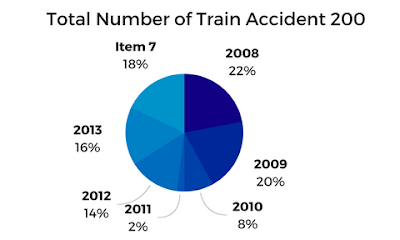# Tricky Solutions For Difficult DI Set: Part 1In this article, we learn how we can calculate DI questions easily by solving most repeatedly asked pattern in our examinations
Guidelines:

• When two diagrams are found they will be interlinked
• These are of three patterns of asking questions
• 1) question directly about diagram 1
• 2) question directly about diagram 2
• 3) question indirectly about both diagrams
• Study the given data to have a rough idea
• Try to find the difference between two diagrams
• Remember the two diagrammatic relations (1st – no of train accidents, 2nd – number of persons) it becomes easy while reading the question.
•  Try to solve DI questions by visual Inspection and mind calculation.

Study the pie-chart and line graph carefully to answer the given questions
The pie-chart shows the percentage of train accidents in different yearsThe line graph shows the number of persons who died in train accidents in various states in different years### Question 1.

The number of persons who died in train accidents in 2013 is how much percent more than the number of persons who died in the train accident in 2011?
a) 143.5%
b) 137.5%
c) 37.5%
d) 127.5%
e) 147.5%
Ans.C
Trick to solve easily:
(2013-2011)/2011 * 100
600/1600 * 100 = 37.5
Explanation:
(2013-2011) = (700-700 + 600-200 + 500-600 + 400-100) = (400-100+300) = 600
2011 = (7+6+2+1) * 100 = 1600
(2013-2011)/2011 = 600/1600 = 37.5%

### Question 2.

What is the average of the number of persons who died in train accidents in 2008 in all states together?
a) 182
b) 290
c) 275
d) 284
e) 307
Ans.C
Trick to solve easily:
Solve by visual Inspection
(1100/4) = 275
Explanation:
(500+300+200+100)/4 = 275

### Question 3.

In which state is the number of persons who died in the train accidents the maximum during the given period?
a) Odisha
b) UP
c) Bihar
d) Only a) and b)
e) Maharashtra
Ans.B.
Trick to solve easily:
Solve by visual Inspection
Explanation:
Odisha = (3+2+7+6+4+5+2)*100 = 2900
Up = (5+6+5+7+6+7+6) * 100 = 4200
Maharashtra = (2+4+1+1+3+4+3) * 100 = 1800
Bihar = (1+3+3+2+5+6+4) * 100 = 2400

### Question 4.

What is the difference between the number of train accidents in 2014 and that in 2012?
a) 5
b) 6
c) 7
d) 8
e) 9
Ans. D.
Trick to solve easily:
(18-14) = 4% of 200 = 8

### Question 5.

What is the ratio of the number of persons who died in train accidents in 2010 to that in 2014?
a) 8 : 7
b) 10 : 9
c) 12 : 11
d) 14 : 13
e) 16 : 15
Ans.E.
Trick to solve easily:
(1+3+5+7) : (2+3+4+6) = 16:15#### What's trending in BankExamsToday

Smart Prep Kit for Banking Exams by Ramandeep Singh - Download here